Definitions of Square Dance Calls and Concepts

Index -->  Plus  |  A1  |  A2  |  C1  |  C2  |  C3A  |  C3B  |  C4  |  NOL  |
Definitions (Text Only) -->  Plus  |  A1  |  A2  |  C1  |  C2  |  C3A  |  C3B  |  C4  |  NOL  |
 Find call:

\$B0lHL2=\$5\$l\$?(B 1/4 Tag \$B\$G(B Very Centers \$B\$,30\$N?M\$H??\$C\$9\$0\$K8~\$+\$\$9g\$C\$F\$\$\$k\$H\$3\$m\$+\$i(B.

1. Very Centers \$B\$H??\$C\$9\$0\$K8~\$+\$\$9g\$C\$F\$\$\$k30\$N?M\$,(B Pass Thru \$B\$r\$7(B;
2. Center 4 \$B\$O(B Cast Off 1/4 and Roll and Spread \$B\$r\$7\$F(B Line \$B\$N(B ends \$B\$K\$J\$j(B, \$BB>\$N?M\$O(B Cross Concentric Vertical 1/2 Tag \$B\$r\$7(B;
3. \$BA40w\$G(B Counter Rotate 1/4 \$B\$r\$7\$^\$9(B.

\$BJ?9T\$J(B Line \$B\$G=*\$o\$j\$^\$9(B. 3 \$B%Q!<%H\$N%3!<%k\$G\$9(B.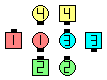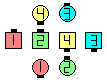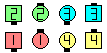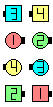Nuclear Reaction\$B\$NA0(B Those Facing Pass Thru\$B\$N8e(B Centers Cast Off 1/4 & Roll & Spread as Ends Cross Concentric Vertical 1/2 Tag\$B\$N8e(B Counter Rotate 1/4\$B\$N8e(B (\$B=*\$o\$j(B)

\$BCm
• Cast Off 1/4 \$B\$O(B Mini-Wave \$B\$^\$?\$O(B Couple \$B\$N\$I\$A\$i\$+\$i\$G\$b9T\$(\$^\$9(B.
• Vertical 1/2 Tag \$B\$G\$OI,\$:1&8*\$GDL\$C\$F\$/\$@\$5\$\$(B.
• Cross Nuclear Reaction [C3B] (Lee Kopman 1988): Nuclear Reaction \$B\$HF1\$8\$G\$9\$,(B, Very Centers \$B\$O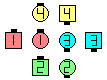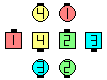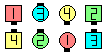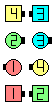Cross Nuclear Reaction\$B\$NA0(B Very Centers and diagonally-facing Outside dancers Jay Walk\$B\$N8e(B Centers Cast Off 1/4 & Roll & Spread as Ends Cross Concentric Vertical 1/2 Tag\$B\$N8e(B Counter Rotate 1/4\$B\$N8e(B (\$B=*\$o\$j(B)

Pass Thru (\$B\$^\$?\$O(B Diagonal Pass Thru) \$B\$r\$9\$k?M\$O(B, Chain Reaction \$B\$N\$H\$-\$HF1\$8\$h\$&\$K(B, \$BF0\$/A0\$K\$*8_\$\$\$K(B point \$B\$9\$Y\$-\$G\$9(B.

anything (Cross) Nuclear Reaction [C3B]: anything call \$B\$r:G8e\$N(B Extend \$B\$NA0\$^\$G9T\$\$(B, (Cross) Nuclear Reaction \$B\$rA4It9T\$\$\$^\$9(B. anything call \$B\$OIaDL(B tagging call \$B\$N(B Scoot Back \$B\$N1~MQ\$G\$9(B.

\$Banything Reaction [C3A].Choreography for Nuclear ReactionComments? Questions? Suggestions?

https://www.ceder.net/def/nuclearreaction.php?level=C3B&language=japan
13-May-2021 17:20:05